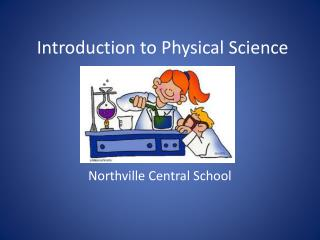# Introduction to Physical Science - PowerPoint PPT PresentationDownload PresentationIntroduction to Physical Science

Introduction to Physical ScienceDownload Presentation## Introduction to Physical Science

- - - - - - - - - - - - - - - - - - - - - - - - - - - E N D - - - - - - - - - - - - - - - - - - - - - - - - - - -
##### Presentation Transcript

1. Introduction to Physical Science Northville Central School

2. Five Steps to the Scientific Method • Question Queen • Hypothesis Helga • Experiment Eats • Data Dry • Conclusion Cereal

3. Scientific Method Rap

4. Major Things to Consider When Writing a Materials and Methods Section: • Materials • Should be complete • List of items with sizes and quantities • Methods • Write so anyone could carry out the procedure (assume the person knows NOTHING!) • List format

5. Metric System • The measurement system used in most countries (not the U.S.) and by ALL scientists. • We will be using the metric system!

6. See Metric System Handout

7. Metric System Measurements • Measurements of: • Weight: gram • Liquids: liter • Length: meter

8. Other “Measurements” • Volume: the amount of space occupied by an object • Volume of a rectangular object (i.e. brick) • V=lwh

9. Other “Measurements” • Mass: a measurement of the quantity of matter in an object; weighing an object

10. Other “Measurements” • Density: mass divided by volume • D=m/v • Water has a density of 1.0 g/mL • Anything less than 1 will float • Anything greater than 1 will sink • Float < 1 < sink